2 views

Could someone please give me a mathematical correct explanation of why a Multilayer Perceptron can solve the XOR problem?

My interpretation of the perceptron is as follows:

A perceptron with two inputs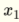and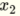has the following linear function and is hence able to solve linear separately problems such as AND and OR.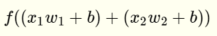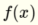is the basic step function.

The way I think of it is that I substitute the two parts withinseparated by the + sign as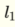and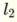I get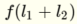which is a line. By applying the step function I get one of the clusters with respect to the input. Which I interpret as one of the spaces separated by that line.

Because the function of an MLP is still linear, how do I interpret this in a mathematical way and more important: Why is it able to solve the XOR problem when it's still linear? Is it because it is interpolating a polynomial?

by (108k points)

The XOr, or “exclusive or”, the problem is a classic problem in ANN research. It is the main problem of using a neural network to predict the outputs of XOr logic gates given two binary inputs. A XOr function should return a true value if the two inputs are not equal and a false value if they are equal. All the possible inputs and their predicted outputs are shown below: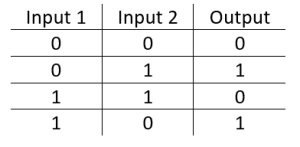Like all ANNs, the perceptron is composed of a network of units, which are analogous to biological neurons. A unit can receive input from other units. In doing so, it takes the sum of all values received and decides whether it is going to forward a signal on to other units to which it is connected. This is called activation. The activation function helps to reduce the sum of input values to a 1 or a 0 (or a value very close to a 1 or 0) in order to represent activation or lack thereof. Another form of unit, known as a bias unit, always activates, typically sending a hardcoded 1 to all units to which it is connected.

Perceptrons include a single layer of input units including one bias unit and a single output unit (as seen below). Here a bias unit is depicted by a dashed circle, while other units are shown as blue circles. There are two non-bias input units representing the two binary input values for XOr. Any number of input units can be included.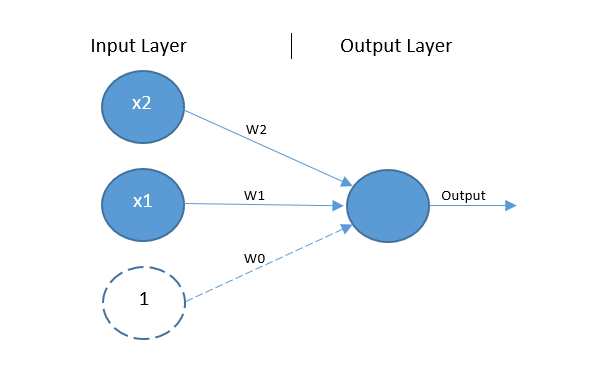https://towardsdatascience.com/perceptrons-logical-functions-and-the-xor-problem-37ca5025790a

If you want to know more about Neural Network visit this Neural Network Tutorial.